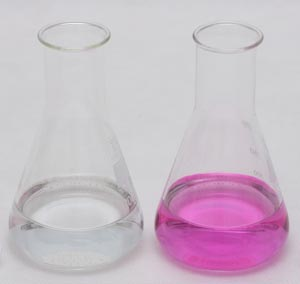# Titration of hydrochloric acid with sodium hydroxide

### general remarks

Determination of hydrochloric acid concentration is probably the most often discussed example of acid-base titration. Both acid and base are strong, which not only makes determination of end point easy (steep part of the curve is long), but also means that calculation of titration curve and equivalence point are pretty straightforward.

### reaction

This is a simple neutralization reaction:

HCl + NaOH → NaCl + H2O

It is worth of noting, that - as we can assume both acid and base to be completely dissociated - net ionic reaction is just

H+ + OH- → H2O

which is the simplest form of neutralization reaction possible.

In the reality every acid and every base - no matter how strong - have some dissociation equilibria described by dissociation constant. In this particular case Ka for HCl is listed as 104 (which means it can be safely neglected) and dissociation constant Kb for NaOH is listed as 0.6 - which means sometimes it has to be taken into account.

### sample size

Depending on the titrant concentration (0.2 M or 0.1 M), and assuming 50 mL burette, aliquot taken for titration should contain about 0.26-0.33 g (0.13-0.16 g) of hydrochloric acid (7-9 or 3.5-4.5 millimoles).

### end point detection

Equivalence point of strong acid titration is usually listed as exactly 7.00. That's not necesarilly the case, as it depends on the solution temperature and ionic strength of the solution, besides, slight hydrolysis of NaOH shifts pH down by about 0.02 unit. Not that it changes much - we are still very close to 7. Thus the best indicator of those listed on pH indicators preparation page is bromothymol blue. However, as we have discussed on the acid-base titration end point detection page, unless we are dealing with a diluted solution (in the range of 0.001 M) we can use almost any indicator that gives observable color change in the pH 4-10 range. Thus we can safely use the most popular phenolphthalein and titrate to the first visible color change.Color change of phenolphthalein during titration - on the left, colorless solution before end point, on the right - pink solution after end point. Note we have to end titration at first sight of color change, before color gets saturated.

### solutions used

To perform titration we will need titrant - 0.2 M or 0.1 M sodium hydroxide solution, indicator - phenolphthalein solution and some amount of distilled water to dilute hydrochloric acid sample.

### procedure

• Pipette aliquot of hydrochloric acid solution into 250mL Erlenmeyer flask.
• Dilute with distilled water to about 100 mL.
• Add 2-3 drops of phenolphthalein solution.
• Titrate with NaOH solution till the first color change.

### result calculation

According to the reaction equation

HCl + NaOH → NaCl + H2O

Hydrochloric acid reacts with sodium hydroxide on the 1:1 basis. That makes calculation especially easy - when we calculate number of moles of NaOH used it will be already number of moles of HCl titrated.

To calculate hydrochloric acid solution concentration use EBAS - stoichiometry calculator. Download determination of hydrochloric acid concentration reaction file, open it with the free trial version of the stoichiometry calculator.

Click button above NaOH in the input frame, enter volume and concentration of the titrant used. Click button. Read number of moles and mass of hydrochloric acid in the titrated sample in the output frame. Click button in the output frame below hydrochloric acid, enter volume of the pipetted sample, read hydrochloric acid concentration.

### sources of errors

Apart from general sources of titration errors, when titrating hydrochloric acid we should pay special attention to titrant. Sodium hydroxide solutions are not stable as they tend to absorb atmospheric carbon dioxide. Hydrochloric acid is much stronger than carbonic acid, so it will slowly expel carbon dioxide from the solution, but initially presence of carbonates will mean that to reach end point we need to add axcess of titrant.